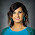## 3.12.2011

### SOHCAHTOA

My instructional coach sent me a link to a great little unit on right triangle trig. If you take a look at it, it's a pretty cool way of grouping students and having them measure angles and sides to find some patterns and ratios.

I also like the visual way of using string and clothes pins to illustrate  the opposite, adjacent, and hypotenuse. I had three students hold the strings of the triangle and stretch all the way across the room. I made the rest of the students sit in the floor inside of the triangle. I made colorful signs and one of them had a bunch of thermometers on it that said 'Degrees'.

I had a student tape the sign to their chest. I placed the student inside the triangle at one of the acute angles. Then I chose three other students to take the signs and pin them to the appropriate string. I asked the rest of the class if their placements were correct and to explain why. On their own, they came up with the fact that the opposite side was the one the student could not touch. Now I had another student become the 'degrees' and move to the other acute angle. Three new students now got the clothes pins and signs and moved them around. This was an important part of the activity because the student who went to move the hypotenuse sign said "Hey, doesn't it stay in the same place?" This was a natural way to discuss where the hypotenuse is, why it is always the same, and why we don't use the right angle as our reference angle.

In my first geometry class, we jumped right from there into the measuring sides and angles activity and it flopped due to the fact that students still weren't understanding exactly where the opposite and adjacent sides were and that they had to look from the perspective of both acute angles. So by the time my second period of geometry came around, I remembered Dave Sladkey's post on kinesthetic right triangle trig. Although I do have a Flip cam, I didn't record it. But I did have students create right triangles with their bodies. I took our handy dandy 'degrees' sign and put it on the floor inside their body triangle at an acute angle. I asked for the opposite, adjacent, and hypotenuse and each person raised their hand accordingly. I did the other acute angle and then rotated to every other group to do the same. Then, (yes we spent a lot of time on figuring this out but what is right triangle trig without knowing that?) I had already drawn a bunch of colorful right triangles all over the board with a theta at random angles to represent the reference angle. I called each student up individually and had them choose any triangle and based on theta, label the sides accordingly. This proved to me that every single student now could correctly identify each side of a right triangle.

Hopefully you have checked out the link by now or this lesson is probably making no sense. From this point, students worked in groups measuring different sized triangles with the same angle and then finding the sine, cosine, and tangent ratios without knowing what they were yet. The idea (I think) was that students would realize their ratios were almost the same for each angle even though their triangle legs were different lengths. Then each group would graph their results and we would have what should be the graphs of sine, cosine, and tangent. Enter great discussion on nonlinear graphs that aren't quadratic and discrete vs. continuous. Maybe I misunderstood the objective of the lesson (READ THE LINK) but the graphs didn't turn out.

The instructions said to have the horizontal axis be degrees 0-90 and the vertical axis to be 0-1 by increments of .1. See below one graph each for sine, cosine, tangent.

But when the students plotted the points, it was just a bunch of scattered clusters of points.

If I connect them, is it going to work out? I'm not sure what the problem is. The students don't know what it's supposed to be yet. I though about giving them some more angles and having them use the calculator to find the value and graph some more points. Maybe we don't have enough data? Or do we have the wrong data? Or have I totally misunderstood the point? Please help.

1.Sorry, but I was following along until it got to the graphing piece. If the point was to make the connection that ratios remain the same for a given angle, regardless of the lengths of each right triangle, then I think a simple table could suffice for generating discussion: triangle #, angle, lengths, sin, cos, tan ratios. But I'm still not clear from the link or above what the goal of the graphing was.

Nice touch in having each student come up to demonstrate understanding.

Paul Hawking
Blog:
The Challenge of Teaching Math
Latest post:
2.3.